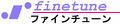Inductance of a straight conductor of circular section

 Straight conductorof circular section Relative permiability and conductanceof popular materials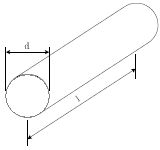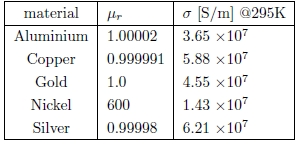wire material: Aluminium Copper Gold Nickel Silver relative permeability (μr) = conductance (μr) = MS/m frequency MHz wire length mm wire diameter mm x T(x) Inductance ≈ nH Reactance ≈ Ω

Formulas used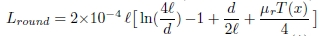[μH]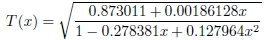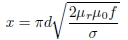where,
d : diamiter of the wire [cm]
f : frequency [Hz]
ℓ : length of the wire [cm]
μr : relative permiability of the conducting material
μ0 : 4 π × 10-7 [H/m]

T(x) is an AC correction from tables , and is asympimated by curve fitting with
a precision of a few percent for 0 ≤ x ≤ 100. For large x, T(x) approaches zero.
Fig.1 Conductor length V.S. Inductance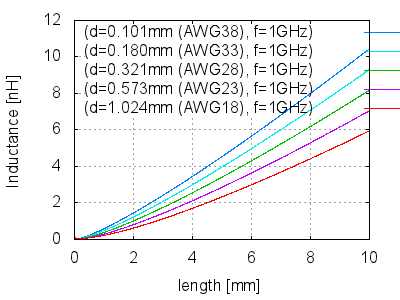Appendix AREFERENCE
1. Brian C. Wadell, "Transmission line design handbook", Artech House, Boston, 1991.
2. F.W. Grouvers, "Inductance calculations", Van Nostrand, New York, 1946.